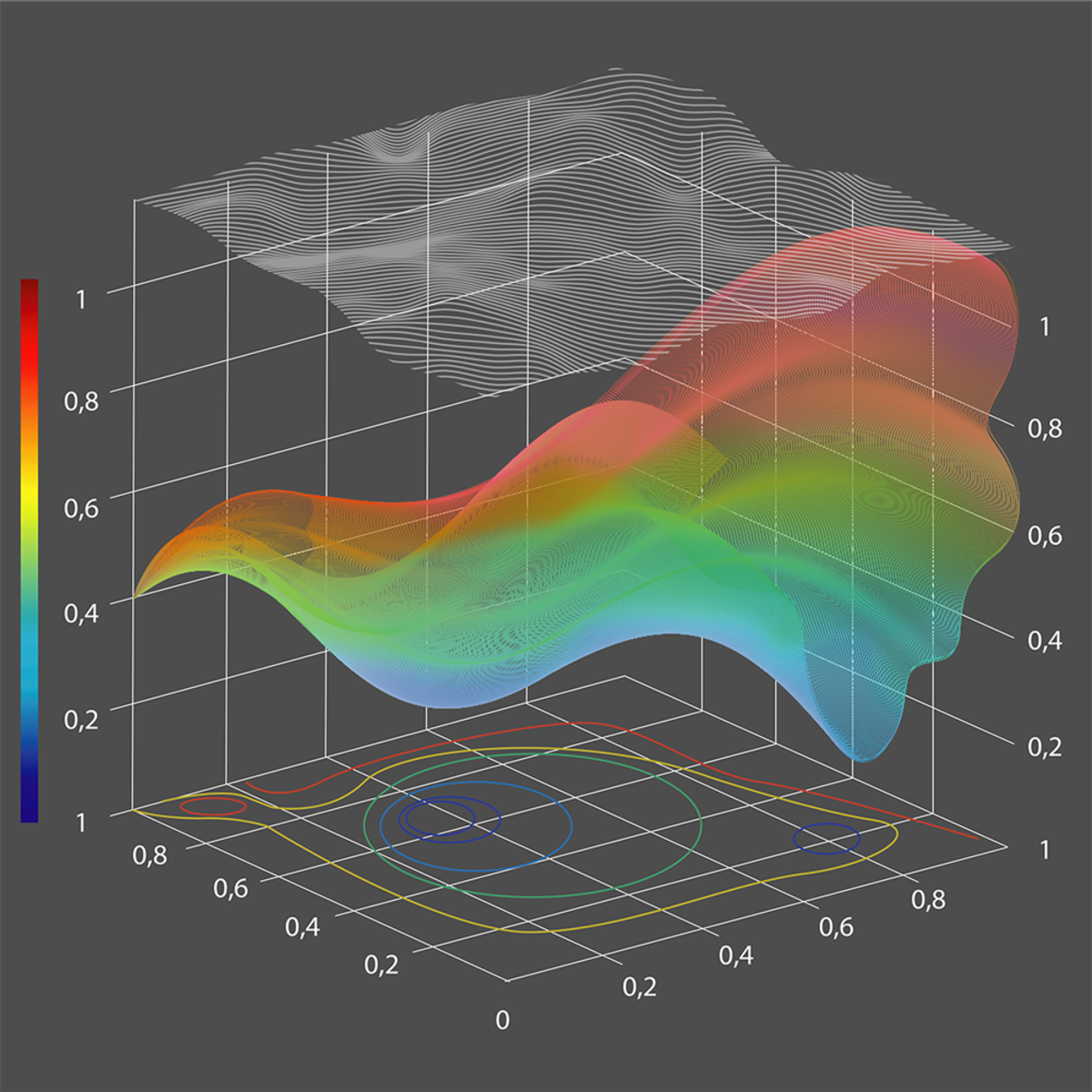Introduction to numerical analysis

Learn Introduction to numerical analysis from National Research University Higher School of Economics. Numerical computations historically play a crucial role in natural sciences and engineering.Free

Description

Featured on: May 18, 2019

It is meant to be an introductory, foundational course in numerical analysis, with the focus on basic ideas. We will review and develop basic characteristics of numerical algorithms (convergence, approximation, stability, computational complexity and so on), and will illustrate them with several classic problems in numerical mathematics. You will also work on implementing abstract mathematical constructions into working prototypes of numerical code. Upon completion of this course, you will have an overview of the main ideas of numerical computing, and will have a solid foundation for reading up on and working with more advanced numerical needs of your specific subject area.